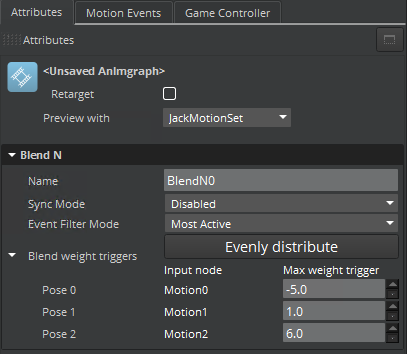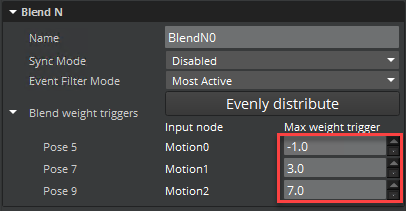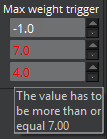Version:

# Blend N Node

The Blend N node accepts up to ten inputs and uses the Weight parameter to determine which inputs to use and their weights. You can specify any type of parameter into the Weight input of a Blend N node.To use the Blend N node

1. In O3DE Editor, choose Tools, Animation Editor.

2. Create a blend tree.

3. Double-click the blend tree node that you created.

4. Select the Anim Graph Palette tab and then select the Blending tab.

5. Drag the Blend N node into the animation graph.1. Connect nodes to the following inputs and output:

• Pose 0 to 9 - Pose inputs. Connect one or more inputs.
• Weight - Input that determines which pose inputs to use and their weights.
• Output Pose - Result of the blended poses.2. Select the Blend N node.

3. For each pose, enter the Max weight trigger in ascending order. Example

If you have three poses, You must specify values in ascending order. The first pose should have the lowest value and the last pose must have the highest.If you enter a series of values in an invalid order, the value box turns red and displays a warning.You can use the Evenly Distribute feature to automatically calculate an even distribution of weights.

To distribute weights using the Evenly Distribute feature

1. In the first input’s Max weight trigger, enter the lowest value.

2. In the last input’s Max weight trigger, enter the highest value.

3. Click Evenly Distribute. This function calculates and evenly spaces the values. Example

You have four inputs. The lowest input is set to 0.0 and the highest input is set to 1.0. Once you click Evenly Distribute, your middle values are automatically calculated to be spaced evenly between 0 and 1. The final values would be 0.0, 0.33, 0.66, and 1.0.

The value of the Weight parameter determines which inputs to blend by its value with respect to the Max weight trigger values. The Weight value naturally falls either before the lowest Max weight trigger values, between two values, or after the highest Max weight trigger value. If it’s lower than the lowest Max weight trigger value, then only that pose is used in the calculation. If it’s higher than the highest Max weight trigger value, then only that pose is used. If it’s between two values, then those two poses are used.

Example Input ports Pose 5, Pose 7, and Pose 9 are connected, with Max weight trigger values of -2.0, 4.0, and 8.0. If the input value is less than or equal to -2.0, then only the port Pose 5 is used to calculate the output pose. If the input is between -2.0 and 4.0, both ports Pose 5 and port Pose 7 are used to calculate the output pose. If the weight is greater than 8.0, then only port Pose 9 is used.

The weight assigned to each value in a pair depends on where the Weight parameter falls with respect to the pose values. It calculates the respective distances and assigns a weight based on its position.

Example The Weight input is set to 0.0. Pose 5 is set to -1.0, and Pose 7 is set to 3.0, which is a difference of 4.0. Because the value of 0.0 is at the 25% point between -1.0 and 3.0, a weight of 0.25 is assigned to Pose 5. The remainder (0.75) is assigned to Pose 7.